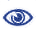# Prague Stringology Conference 2011

Computing Abelian Periods in Words

 Abstract: In the last couple of years many works have been devoted to Abelian complexity of words. Recently, Constantinescu and Ilie (Bulletin EATCS 89, 167–170, 2006) introduced the notion of Abelian period. We show that a word w of length n over an alphabet of size σ can have Θ(n2) distinct Abelian periods. However, to the best of our knowledge, no efficient algorithm is known for computing these periods. The Brute-Force algorithm computes all the Abelian periods either in time O(n3 × σ) using O(σ) space or in time O(n2 × σ) using O(n × σ) space. We present an off-line algorithm running in time O(n2 × σ) using O(n + σ) space, thus improving the space complexity. This algorithm is based on a select function. We then present on-line algorithms that also enable to compute all the Abelian periods of all the prefixes of w. Experimental results show that the new off-line algorithm is faster than the Brute-Force one. Moreover, in most cases, one on-line algorithm, though having a worst case time complexity, is also faster than the Brute-Force one.

 Download paper:PostScript PDF BibTeX reference
 Download presentation: